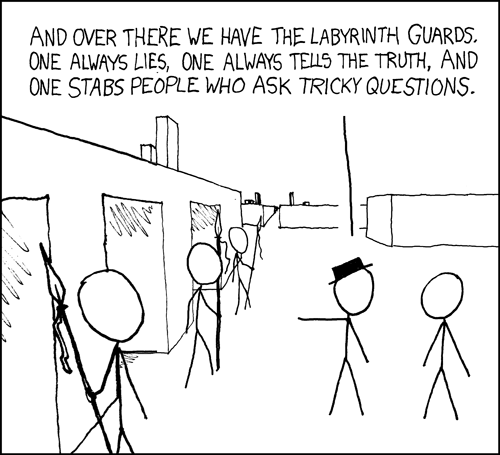# Facts and puzzles.

• Posted on: 12 October 2020
Block:
Term:
Year:
LA Code:
Code:
Room:
Learning Area:
Levels:
4

In this class we will do 15 minutes of basic math facts from :

• Bedmas
• timetables
• fractions
• units of measure

, then we will work on a logic puzzle, learning mathematical concepts through puzzles.